CI Core Engine

Compression-ignition engine from intake to exhaust port

• Library:
• Powertrain Blockset / Propulsion / Combustion Engine Components / Core Engine

•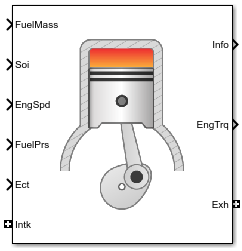Description

The CI Core Engine block implements a compression-ignition (CI) engine from intake to the exhaust port. You can use the block for hardware-in-the-loop (HIL) engine control design or vehicle-level fuel economy and performance simulations.

The CI Core Engine block calculates:

• Brake torque

• Exhaust temperature

• Air-fuel ratio (AFR)

• Fuel rail pressure

• Engine-out (EO) exhaust emissions:

• Hydrocarbon (HC)

• Carbon monoxide (CO)

• Nitric oxide and nitrogen dioxide (NOx)

• Carbon dioxide (CO2)

• Particulate matter (PM)

Air Mass Flow

To calculate the air mass flow, the compression-ignition (CI) engine uses the CI Engine Speed-Density Air Mass Flow Model. The speed-density model uses the speed-density equation to calculate the engine air mass flow, relating the engine intake port mass flow to the intake manifold pressure, intake manifold temperature, and engine speed.

Brake Torque

To calculate the engine torque, you can configure the block to use either of these torque models.

Brake Torque ModelDescription
CI Engine Torque Structure Model

The CI core engine torque structure model determines the engine torque by reducing the maximum engine torque potential as these engine conditions vary from nominal:

• Start of injection (SOI) timing

• Exhaust back-pressure

• Burned fuel mass

• Intake manifold gas pressure, temperature, and oxygen percentage

• Fuel rail pressure

To account for the effect of post-inject fuel on torque, the model uses a calibrated torque offset table.

CI Engine Simple Torque Model

For the simple engine torque calculation, the CI engine uses a torque lookup table map that is a function of engine speed and injected fuel mass.

Fuel Flow

In the CI Core Engine and CI Controller blocks, you can represent multiple injections with the start of injection (SOI) and fuel mass inputs to the model. To specify the type of injection, use the Fuel mass injection type identifier parameter.

Type of InjectionParameter Value

Pilot

0

Main

1

Post

2

Passed

3

The model considers Passed fuel injections and fuel injected later than a threshold to be unburned fuel. Use the parameter to specify the threshold.

To calculate the engine fuel mass flow, the CI Core Engine block uses fuel mass flow delivered by the injectors and the engine airflow.

${\stackrel{˙}{m}}_{fuel}=\frac{N\cdot {N}_{cyl}}{Cps\left(\frac{60s}{\mathrm{min}}\right)\left(\frac{1000mg}{g}\right)}\sum {m}_{fuel,inj}$

To calculate the fuel economy for high-fidelity models, the block uses the volumetric fuel flow.

${Q}_{fuel}=\frac{{\stackrel{˙}{m}}_{fuel}}{\left(\frac{1000kg}{{m}^{3}}\right)S{g}_{fuel}}$

The equation uses these variables.

 ${\stackrel{˙}{m}}_{fuel}$ Fuel mass flow, g/s mfuel,inj Fuel mass per injection $Cps$ Crankshaft revolutions per power stroke, rev/stroke ${N}_{cyl}$ Number of engine cylinders N Engine speed, rpm Qfuel Volumetric fuel flow Sgfuel Specific gravity of fuel

The block uses the internal signal FlwDir to track the direction of the flow.

Air-Fuel Ratio

To calculate the air-fuel (AFR) ratio, the CI Core Engine and SI Core Engine blocks implement this equation.

$AFR=\frac{{\stackrel{˙}{m}}_{air}}{{\stackrel{˙}{m}}_{fuel}}$

The CI Core Engine uses this equation to calculate the relative AFR.

$\lambda =\frac{AFR}{AF{R}_{s}}$

To calculate the exhaust gas recirculation (EGR), the blocks implement this equation. The calculation expresses the EGR as a percent of the total intake port flow.

$EG{R}_{pct}=100\frac{{\stackrel{˙}{m}}_{intk,b}}{{\stackrel{˙}{m}}_{intk}}=100{y}_{intk,b}$

The equations use these variables.

 $AFR$ Air-fuel ratio AFRs Stoichiometric air-fuel ratio ${\stackrel{˙}{m}}_{intk}$ Engine air mass flow ${\stackrel{˙}{m}}_{fuel}$ Fuel mass flow λ Relative AFR yintk,b Intake burned mass fraction EGRpct EGR percent ${\stackrel{˙}{m}}_{intk,b}$ Recirculated burned gas mass flow rate

Exhaust Temperature

The exhaust temperature calculation depends on the torque model. For both torque models, the block implements lookup tables.

Torque Model

Description

Equations

Simple Torque Lookup

Exhaust temperature lookup table is a function of the injected fuel mass and engine speed.

${T}_{exh}={f}_{Texh}\left(F,N\right)$

Torque Structure

The nominal exhaust temperature, Texhnom, is a product of these exhaust temperature efficiencies:

• SOI timing

• Intake manifold gas pressure

• Intake manifold gas temperature

• Intake manifold gas oxygen percentage

• Fuel rail pressure

• Optimal temperature

The exhaust temperature, Texhnom, is offset by a post temperature effect, ΔTpost, that accounts for post and late injections during the expansion and exhaust strokes.

$\begin{array}{l}{T}_{exhnom}=SO{I}_{exhteff}MA{P}_{exhteff}MA{T}_{exhteff}O2{p}_{exhteff}FUEL{P}_{exhteff}Tex{h}_{opt}\\ {T}_{exh}={T}_{exhnom}+\Delta {T}_{post}\\ \\ SO{I}_{exhteff}={f}_{SO{I}_{exhteff}}\left(\Delta SOI,N\right)\\ MA{P}_{exhteff}={f}_{MA{P}_{exhteff}}\left(MA{P}_{ratio},\lambda \right)\\ MA{T}_{exhteff}={f}_{MA{T}_{exhteff}}\left(\Delta MAT,N\right)\\ O2{p}_{exhteff}={f}_{O2{p}_{exhteff}}\left(\Delta O2p,N\right)\\ Tex{h}_{opt}={f}_{Texh}\left(F,N\right)\end{array}$

The equations use these variables.

 F Compression stroke injected fuel mass N Engine speed Texh Exhaust manifold gas temperature Texhopt Optimal exhaust manifold gas temperature ΔTpost Post injection temperature effect Texhnom Nominal exhaust temperature SOIexhteff Main SOI exhaust temperature efficiency multiplier ΔSOI Main SOI timing relative to optimal timing MAPexheff Intake manifold gas pressure exhaust temperature efficiency multiplier MAPratio Intake manifold gas pressure ratio relative to optimal pressure ratio λ Intake manifold gas lambda MATexheff Intake manifold gas temperature exhaust temperature efficiency multiplier ΔMAT Intake manifold gas temperature relative to optimal temperature O2Pexheff Intake manifold gas oxygen exhaust temperature efficiency multiplier ΔO2P Intake gas oxygen percent relative to optimal FUELPexheff Fuel rail pressure exhaust temperature efficiency multiplier ΔFUELP Fuel rail pressure relative to optimal

EO Exhaust Emissions

The block calculates these engine-out (EO) exhaust emissions:

• Hydrocarbon (HC)

• Carbon monoxide (CO)

• Nitric oxide and nitrogen dioxide (NOx)

• Carbon dioxide (CO2)

• Particulate matter (PM)

The exhaust temperature determines the specific enthalpy.

${h}_{exh}=C{p}_{exh}{T}_{exh}$

The exhaust mass flow rate is the sum of the intake port air mass flow and the fuel mass flow.

${\stackrel{˙}{m}}_{exh}={\stackrel{˙}{m}}_{intake}+{\stackrel{˙}{m}}_{fuel}$

To calculate the exhaust emissions, the block multiplies the emission mass fraction by the exhaust mass flow rate. To determine the emission mass fractions, the block uses lookup tables that are functions of the engine torque and speed.

$\begin{array}{l}{y}_{exh,i}={f}_{i_frac}\left({T}_{brake},N\right)\\ {\stackrel{˙}{m}}_{exh,i}={\stackrel{˙}{m}}_{exh}{y}_{exh,i}\end{array}$

The fraction of air and fuel entering the intake port, injected fuel, and stoichiometric AFR determine the air mass fraction that exits the exhaust.

${y}_{exh,air}=\mathrm{max}\left[{y}_{in,air}-\frac{{\stackrel{˙}{m}}_{fuel}+{y}_{in,fuel}{\stackrel{˙}{m}}_{intake}}{{\stackrel{˙}{m}}_{fuel}+{\stackrel{˙}{m}}_{intake}}AF{R}_{s}\right]$

If the engine is operating at the stoichiometric or fuel rich AFR, no air exits the exhaust. Unburned hydrocarbons and burned gas comprise the remainder of the exhaust gas. This equation determines the exhaust burned gas mass fraction.

${y}_{exh,b}=\mathrm{max}\left[\left(1-{y}_{exh,air}-{y}_{exh,HC}\right),0\right]$

The equations use these variables.

 ${T}_{exh}$ Engine exhaust temperature ${h}_{exh}$ Exhaust manifold inlet-specific enthalpy $C{p}_{exh}$ Exhaust gas specific heat ${\stackrel{˙}{m}}_{intk}$ Intake port air mass flow rate ${\stackrel{˙}{m}}_{fuel}$ Fuel mass flow rate ${\stackrel{˙}{m}}_{exh}$ Exhaust mass flow rate ${y}_{in,fuel}$ Intake fuel mass fraction yexh,i Exhaust mass fraction for i = CO2, CO, HC, NOx, air, burned gas, and PM ${\stackrel{˙}{m}}_{exh,i}$ Exhaust mass flow rate for i = CO2, CO, HC, NOx, air, burned gas, and PM Tbrake Engine brake torque N Engine speed yexh,air Exhaust air mass fraction yexh,b Exhaust air burned mass fraction

Power Accounting

For the power accounting, the block implements equations that depend on Torque model.

When you set Torque model to Simple Torque Lookup, the block implements these equations.

Bus Signal DescriptionEquations

PwrInfo

PwrTrnsfrd — Power transferred between blocks

• Positive signals indicate flow into block

• Negative signals indicate flow out of block

PwrIntkHeatFlw

Intake heat flow

${\stackrel{˙}{m}}_{intk}{h}_{intk}$

PwrExhHeatFlw

Exhaust heat flow

$-{\stackrel{˙}{m}}_{exh}{h}_{exh}$

PwrCrkshftCrankshaft power

$-{T}_{brake}\omega$

PwrNotTrnsfrd — Power crossing the block boundary, but not transferred

• Positive signals indicate an input

• Negative signals indicate a loss

PwrFuel

Fuel input power

${\stackrel{˙}{m}}_{fuel}LHV$

PwrLoss

All losses

${T}_{brake}\omega -{\stackrel{˙}{m}}_{fuel}LHV-{\stackrel{˙}{m}}_{intk}{h}_{intk}+{\stackrel{˙}{m}}_{exh}{h}_{exh}$

PwrStored — Stored energy rate of change

• Positive signals indicate an increase

• Negative signals indicate a decrease

Not used

When you set Torque model to Torque Structure, the block implements these equations.

Bus Signal DescriptionEquations

PwrInfo

PwrTrnsfrd — Power transferred between blocks

• Positive signals indicate flow into block

• Negative signals indicate flow out of block

PwrIntkHeatFlw

Intake heat flow

${\stackrel{˙}{m}}_{intk}{h}_{intk}$

PwrExhHeatFlw

Exhaust heat flow

$-{\stackrel{˙}{m}}_{exh}{h}_{exh}$

PwrCrkshftCrankshaft power

$-{T}_{brake}\omega$

PwrNotTrnsfrd — Power crossing the block boundary, but not transferred

• Positive signals indicate an input

• Negative signals indicate a loss

PwrFuel

Fuel input power

${\stackrel{˙}{m}}_{fuel}LHV$

PwrFricLoss

Friction loss

$-{T}_{fric}\omega$

PwrPumpLoss

Pumping loss

$-{T}_{pump}\omega$

PwrHeatTrnsfrLoss

Heat transfer loss

${T}_{brake}\omega -{\stackrel{˙}{m}}_{fuel}LHV-{\stackrel{˙}{m}}_{intk}{h}_{intk}+{\stackrel{˙}{m}}_{exh}{h}_{exh}+{T}_{fric}\omega +{T}_{pump}\omega$

PwrStored — Stored energy rate of change

• Positive signals indicate an increase

• Negative signals indicate a decrease

Not used

 hexh Exhaust manifold inlet-specific enthalpy hintk Intake port specific enthalpy ${\stackrel{˙}{m}}_{intk}$ Intake port air mass flow rate ${\stackrel{˙}{m}}_{fuel}$ Fuel mass flow rate ${\stackrel{˙}{m}}_{exh}$ Exhaust mass flow rate ω Engine speed Tbrake Brake torque Tpump Engine pumping work offset to inner torque Tfric Engine friction torque LHV Fuel lower heating value

Ports

Input

expand all

Fuel mass per injection, mfuel,inj, in mg per injection.

Fuel injection timing, SOI, in degrees crank angle after top dead center (degATDC). First vector value, Soi(1), is main injection timing.

Dependencies

To enable this parameter, for Torque model, select Torque Structure.

Engine speed, N, in rpm.

Fuel rail pressure, FUELP, in MPa.

Dependencies

To enable this parameter, for Torque model, select Torque Structure.

Engine cooling temperature, Tcoolant, in K.

Dependencies

To enable this parameter, for Torque model, select Torque Structure.

Bus containing the upstream:

• Prs — Pressure, in Pa

• Temp — Temperature, in K

• Enth — Specific enthalpy, in J/kg

• MassFrac — Intake port mass fractions, dimensionless. Exhaust gas recirculation (EGR) mass flow at the intake port is burned gas.

Specifically, a bus with these mass fractions:

• O2MassFrac — Oxygen

• N2MassFrac — Nitrogen

• UnbrndFuelMassFrac — Unburned fuel

• CO2MassFrac — Carbon dioxide

• H2OMassFrac — Water

• COMassFrac — Carbon monoxide

• NOMassFrac — Nitric oxide

• NO2MassFrac — Nitrogen dioxide

• NOxMassFrac — Nitric oxide and nitrogen dioxide

• PmMassFrac — Particulate matter

• AirMassFrac — Air

• BrndGasMassFrac — Burned gas

Bus containing the exhaust:

• Prs — Pressure, in Pa

• Temp — Temperature, in K

• Enth — Specific enthalpy, in J/kg

• MassFrac — Exhaust port mass fractions, dimensionless.

Specifically, a bus with these mass fractions:

• O2MassFrac — Oxygen

• N2MassFrac — Nitrogen

• UnbrndFuelMassFrac — Unburned fuel

• CO2MassFrac — Carbon dioxide

• H2OMassFrac — Water

• COMassFrac — Carbon monoxide

• NOMassFrac — Nitric oxide

• NO2MassFrac — Nitrogen dioxide

• NOxMassFrac — Nitric oxide and nitrogen dioxide

• PmMassFrac — Particulate matter

• AirMassFrac — Air

• BrndGasMassFrac — Burned gas

Output

expand all

Bus signal containing these block calculations.

SignalDescriptionVariableUnits

IntkGasMassFlw

Engine intake air mass flow.

${\stackrel{˙}{m}}_{air}$

kg/s

IntkAirMassFlw

Engine intake port mass flow.

${\stackrel{˙}{m}}_{intk}$

kg/s

NrmlzdAirChrg

Engine load (that is, normalized cylinder air mass) corrected for final steady-state cam phase angles

$L$N/A

Afr

Air-fuel ratio at engine exhaust port

$AFR$N/A

FuelMassFlw

Fuel flow into engine

${\stackrel{˙}{m}}_{fuel}$kg/s

FuelVolFlw

Volumetric fuel flow

Qfuel

m3/s

ExhManGasTemp

Exhaust gas temperature at exhaust manifold inlet

${T}_{exh}$K

EngTrq

Engine brake torque

${T}_{brake}$N·m

EngSpd

Engine speed

$N$rpm

IntkCamPhase

Intake cam phaser angle

${\phi }_{ICP}$ i

ExhCamPhase

Exhaust cam phaser angle

${\phi }_{ECP}$

degrees crank retard

CrkAng

Engine crankshaft absolute angle

$\underset{0}{\overset{\left(360\right)Cps}{\int }}EngSpd\frac{180}{30}d\theta$

where $Cps$ is crankshaft revolutions per power stroke

degrees crank angle

EgrPct

EGR percent

EGRpct

N/A

EoAir

EO air mass flow rate

${\stackrel{˙}{m}}_{exh}$

kg/s

EoBrndGas

EO burned gas mass flow rate

yexh,b

kg/s

EoHC

EO hydrocarbon emission mass flow rate

yexh,HC

kg/s

EoCO

EO carbon monoxide emission mass flow rate

yexh,CO

kg/s

EoNOx

EO nitric oxide and nitrogen dioxide emissions mass flow rate

yexh,NOx

kg/s

EoCO2

EO carbon dioxide emission mass flow rate

yexh,CO2

kg/s

EoPm

EO particulate matter emission mass flow rate

yexh,PM

kg/s

PwrInfoPwrTrnsfrd

PwrIntkHeatFlw

Intake heat flow

${\stackrel{˙}{m}}_{intk}{h}_{intk}$

W
PwrExhHeatFlw

Exhaust heat flow

$-{\stackrel{˙}{m}}_{exh}{h}_{exh}$

W
PwrCrkshftCrankshaft power

$-{T}_{brake}\omega$

W
PwrNotTrnsfrdPwrFuel

Fuel input power

${\stackrel{˙}{m}}_{fuel}LHV$

W
PwrLoss

For Torque model set to Simple Torque Lookup:

All losses

${T}_{brake}\omega -{\stackrel{˙}{m}}_{fuel}LHV-{\stackrel{˙}{m}}_{intk}{h}_{intk}+{\stackrel{˙}{m}}_{exh}{h}_{exh}$

W
PwrFricLoss

For Torque model set to Torque Structure:

Friction loss

$-{T}_{fric}\omega$

W
PwrPumpLoss

For Torque model set to Torque Structure:

Pumping loss

$-{T}_{pump}\omega$

W
PwrHeatTrnsfrLoss

For Torque model set to Torque Structure:

Heat transfer loss

${T}_{brake}\omega -{\stackrel{˙}{m}}_{fuel}LHV-{\stackrel{˙}{m}}_{intk}{h}_{intk}+{\stackrel{˙}{m}}_{exh}{h}_{exh}+{T}_{fric}\omega +{T}_{pump}\omega$

W
PwrStoredNot used

Engine brake torque, ${T}_{brake}$, in N·m.

Bus containing:

• MassFlwRate — Intake port mass flow rate, in kg/s

• HeatFlwRate — Intake port heat flow rate, in J/s

• ExhManGasTemp — Intake port temperature, in K

• MassFrac — Intake port mass fractions, dimensionless.

Specifically, a bus with these mass fractions:

• O2MassFrac — Oxygen

• N2MassFrac — Nitrogen

• UnbrndFuelMassFrac — Unburned fuel

• CO2MassFrac — Carbon dioxide

• H2OMassFrac — Water

• COMassFrac — Carbon monoxide

• NOMassFrac — Nitric oxide

• NO2MassFrac — Nitrogen dioxide

• NOxMassFrac — Nitric oxide and nitrogen dioxide

• PmMassFrac — Particulate matter

• AirMassFrac — Air

• BrndGasMassFrac — Burned gas

Bus containing:

• MassFlwRate — Exhaust port mass flow rate, in kg/s

• HeatFlwRate — Exhaust heat flow rate, in J/s

• ExhManGasTemp — Exhaust port temperature, in K

• MassFrac — Exhaust port mass fractions, dimensionless.

Specifically, a bus with these mass fractions:

• O2MassFrac — Oxygen

• N2MassFrac — Nitrogen

• UnbrndFuelMassFrac — Unburned fuel

• CO2MassFrac — Carbon dioxide

• H2OMassFrac — Water

• COMassFrac — Carbon monoxide

• NOMassFrac — Nitric oxide

• NO2MassFrac — Nitrogen dioxide

• NOxMassFrac — Nitric oxide and nitrogen dioxide

• PmMassFrac — Particulate matter

• AirMassFrac — Air

• BrndGasMassFrac — Burned gas

Parameters

expand all

Block Options

To calculate the engine torque, you can configure the block to use either of these torque models.

Brake Torque ModelDescription
CI Engine Torque Structure Model

The CI core engine torque structure model determines the engine torque by reducing the maximum engine torque potential as these engine conditions vary from nominal:

• Start of injection (SOI) timing

• Exhaust back-pressure

• Burned fuel mass

• Intake manifold gas pressure, temperature, and oxygen percentage

• Fuel rail pressure

To account for the effect of post-inject fuel on torque, the model uses a calibrated torque offset table.

CI Engine Simple Torque Model

For the simple engine torque calculation, the CI engine uses a torque lookup table map that is a function of engine speed and injected fuel mass.

Air

Number of engine cylinders, ${N}_{cyl}$.

Standard air temperature, Tstd, in K.

Crankshaft revolutions per power stroke, $Cps$, in rev/stroke.

Displaced volume, ${V}_{d}$, in m^3.

Ideal gas constant, ${R}_{air}$, in J/(kg·K).

Standard air pressure, ${P}_{std}$, in Pa.

The volumetric efficiency lookup table is a function of the intake manifold absolute pressure at intake valve closing (IVC) and engine speed

${\eta }_{v}={f}_{{\eta }_{v}}\left(MAP,N\right)$

where:

• ${\eta }_{v}$ is engine volumetric efficiency, dimensionless.

• MAP is intake manifold absolute pressure, in KPa.

• N is engine speed, in rpm.Intake manifold pressure breakpoints for speed-density volumetric efficiency lookup table, in KPa.

Engine speed breakpoints for speed-density volumetric efficiency lookup table, in rpm.

Torque

Torque - Simple Torque Lookup

For the simple torque lookup table model, the CI engine uses a lookup table is a function of engine speed and injected fuel mass, ${T}_{brake}={f}_{Tnf}\left(F,N\right)$, where:

• Tq = Tbrake is engine brake torque after accounting for engine mechanical and pumping friction effects, in N·m.

• F is injected fuel mass, in mg per injection.

• N is engine speed, in rpm.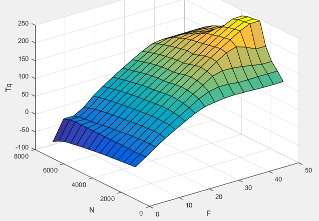Dependencies

To enable this parameter, for Torque model, select Simple Torque Lookup.

Torque table fuel mass per injection breakpoints, in mg per injection.

Dependencies

To enable this parameter, for Torque model, select Simple Torque Lookup.

Engine speed breakpoints, in rpm.

Dependencies

To enable this parameter, for Torque model, select Simple Torque Lookup.

Torque - Torque Structure

Fuel mass per injection breakpoints, in mg per injection.

Dependencies

To enable this parameter, for Torque model, select Torque Structure.

Engine speed breakpoints, in rpm.

Dependencies

To enable this parameter, for Torque model, select Torque Structure.

The optimal main start of injection (SOI) timing lookup table, ƒSOIc, is a function of the engine speed and injected fuel mass, SOIc = ƒSOIc(F,N), where:

• SOIc is optimal SOI timing, in degATDC.

• F is compression stroke injected fuel mass, in mg per injection.

• N is engine speed, in rpm.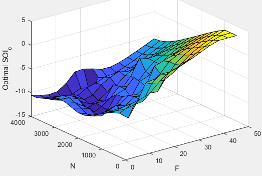Dependencies

To enable this parameter, for Torque model, select Torque Structure.

The optimal intake manifold gas pressure lookup table, ƒMAP, is a function of the engine speed and injected fuel mass, MAP = ƒMAP(F,N), where:

• MAP is optimal intake manifold gas pressure, in Pa.

• F is compression stroke injected fuel mass, in mg per injection.

• N is engine speed, in rpm.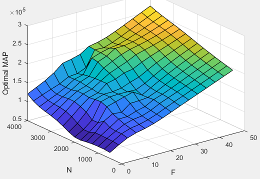Dependencies

To enable this parameter, for Torque model, select Torque Structure.

The optimal exhaust manifold gas pressure lookup table, ƒEMAP, is a function of the engine speed and injected fuel mass, EMAP = ƒEMAP(F,N), where:

• EMAP is optimal exhaust manifold gas pressure, in Pa.

• F is compression stroke injected fuel mass, in mg per injection.

• N is engine speed, in rpm.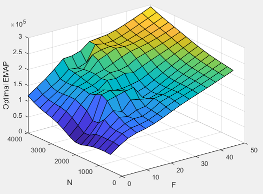Dependencies

To enable this parameter, for Torque model, select Torque Structure.

The optimal intake manifold gas temperature lookup table, ƒMAT, is a function of the engine speed and injected fuel mass, MAT = ƒMAT(F,N), where:

• MAT is optimal intake manifold gas temperature, in K.

• F is compression stroke injected fuel mass, in mg per injection.

• N is engine speed, in rpm.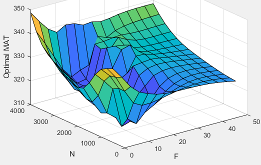Dependencies

To enable this parameter, for Torque model, select Torque Structure.

The optimal intake gas oxygen percent lookup table, ƒO2, is a function of the engine speed and injected fuel mass, O2PCT = ƒO2(F,N), where:

• O2PCT is optimal intake gas oxygen, in percent.

• F is compression stroke injected fuel mass, in mg per injection.

• N is engine speed, in rpm.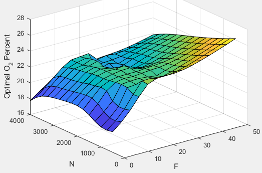Dependencies

To enable this parameter, for Torque model, select Torque Structure.

The optimal fuel rail pressure lookup table, ƒfuelp, is a function of the engine speed and injected fuel mass, FUELP = ƒfuelp(F,N), where:

• FUELP is optimal fuel rail pressure, in MPa.

• F is compression stroke injected fuel mass, in mg per injection.

• N is engine speed, in rpm.Dependencies

To enable this parameter, for Torque model, select Torque Structure.

The optimal gross indicated mean effective pressure lookup table, ƒimepg, is a function of the engine speed and injected fuel mass, IMEPG = ƒimepg(F,N), where:

• IMEPG is optimal gross indicated mean effective pressure, in Pa.

• F is compression stroke injected fuel mass, in mg per injection.

• N is engine speed, in rpm.Dependencies

To enable this parameter, for Torque model, select Torque Structure.

The optimal friction mean effective pressure lookup table, ƒfmep, is a function of the engine speed and injected fuel mass, FMEP = ƒfmep(F,N), where:

• FMEP is optimal friction mean effective pressure, in Pa.

• F is compression stroke injected fuel mass, in mg per injection.

• N is engine speed, in rpm.Dependencies

To enable this parameter, for Torque model, select Torque Structure.

The optimal pumping mean effective pressure lookup table, ƒpmep, is a function of the engine speed and injected fuel mass, PMEP = ƒpmep(F,N), where:

• PMEP is optimal pumping mean effective pressure, in Pa.

• F is compression stroke injected fuel mass, in mg per injection.

• N is engine speed, in rpm.Dependencies

To enable this parameter, for Torque model, select Torque Structure.

Friction multiplier as a function of temperature, dimensionless.

Dependencies

To enable this parameter, for Torque model, select Torque Structure.

Friction multiplier temperature breakpoints, in K.

Dependencies

To enable this parameter, for Torque model, select Torque Structure.

The main start of injection (SOI) timing efficiency multiplier lookup table, ƒSOIeff, is a function of the engine speed and main SOI timing relative to optimal timing, SOIeff = ƒSOIeff(ΔSOI,N), where:

• SOIeff is main SOI timing efficiency multiplier, dimensionless.

• ΔSOI is main SOI timing relative to optimal timing, in degBTDC.

• N is engine speed, in rpm.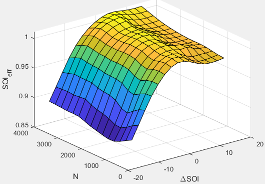Dependencies

To enable this parameter, for Torque model, select Torque Structure.

Main start of injection timing relative to optimal timing breakpoints, in degBTDC.

Dependencies

To enable this parameter, for Torque model, select Torque Structure.

The intake manifold gas pressure efficiency multiplier lookup table, ƒMAPeff, is a function of the intake manifold gas pressure ratio relative to optimal pressure ratio and lambda, MAPeff = ƒMAPeff(MAPratio,λ), where:

• MAPeff is intake manifold gas pressure efficiency multiplier, dimensionless.

• MAPratio is intake manifold gas pressure ratio relative to optimal pressure ratio, dimensionless.

• λ is intake manifold gas lambda, dimensionless.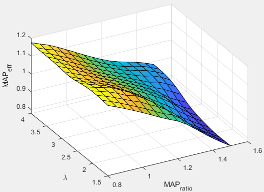Dependencies

To enable this parameter, for Torque model, select Torque Structure.

Intake manifold gas pressure ratio relative to optimal pressure ratio breakpoints, dimensionless.

Dependencies

To enable this parameter, for Torque model, select Torque Structure.

Intake manifold gas lambda breakpoints, dimensionless.

Dependencies

To enable this parameter, for Torque model, select Torque Structure.

The intake manifold gas temperature efficiency multiplier lookup table, ƒMATeff, is a function of the engine speed and intake manifold gas temperature relative to optimal temperature, MATeff = ƒMATeff(ΔMAT,N), where:

• MATeff is intake manifold gas temperature efficiency multiplier, dimensionless.

• ΔMAT is intake manifold gas temperature relative to optimal temperature, in K.

• N is engine speed, in rpm.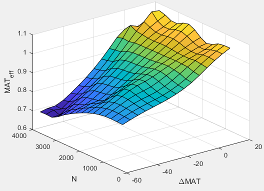Dependencies

To enable this parameter, for Torque model, select Torque Structure.

Intake manifold gas temperature relative to optimal gas temperature breakpoints, in K.

Dependencies

To enable this parameter, for Torque model, select Torque Structure.

The intake manifold gas oxygen efficiency multiplier lookup table, ƒO2Peff, is a function of the engine speed and intake manifold gas oxygen percent relative to optimal, O2Peff = ƒO2Peff(ΔO2P,N), where:

• O2Peff is intake manifold gas oxygen efficiency multiplier, dimensionless.

• ΔO2P is intake gas oxygen percent relative to optimal, in percent.

• N is engine speed, in rpm.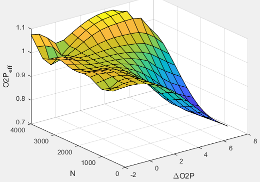Dependencies

To enable this parameter, for Torque model, select Torque Structure.

Intake gas oxygen percent relative to optimal breakpoints, in percent.

Dependencies

To enable this parameter, for Torque model, select Torque Structure.

The fuel rail pressure efficiency multiplier lookup table, ƒFUELPeff, is a function of the engine speed and fuel rail pressure relative to optimal breakpoints, FUELPeff = ƒFUELPeff(ΔFUELP,N), where:

• FUELPeff is fuel rail pressure efficiency multiplier, dimensionless.

• ΔFUELP is fuel rail pressure relative to optimal, in MPa.

• N is engine speed, in rpm.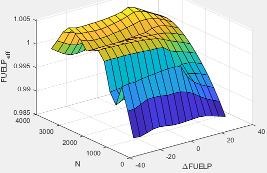Dependencies

To enable this parameter, for Torque model, select Torque Structure.

Fuel rail pressure relative to optimal breakpoints, in MPa.

Dependencies

To enable this parameter, for Torque model, select Torque Structure.

Fuel mass injection type identifier, dimensionless.

In the CI Core Engine and CI Controller blocks, you can represent multiple injections with the start of injection (SOI) and fuel mass inputs to the model. To specify the type of injection, use the Fuel mass injection type identifier parameter.

Type of InjectionParameter Value

Pilot

0

Main

1

Post

2

Passed

3

The model considers Passed fuel injections and fuel injected later than a threshold to be unburned fuel. Use the parameter to specify the threshold.

Dependencies

To enable this parameter, for Torque model, select Torque Structure.

The indicated mean effective pressure post inject correction lookup table, ƒIMEPpost, is a function of the engine speed and fuel rail pressure relative to optimal breakpoints, ΔIMEPpost = ƒIMEPpost(ΔSOIpost,Fpost), where:

• ΔIMEPpost is indicated mean effective pressure post inject correction, in Pa.

• ΔSOIpost is indicated mean effective pressure post inject start of inject timing centroid, in degATDC.

• Fpost is indicated mean effective pressure post inject mass sum, in mg per injection.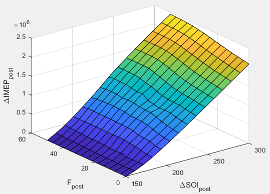Dependencies

To enable this parameter, for Torque model, select Torque Structure.

Indicated mean effective pressure post inject mass sum breakpoints, in mg per injection.

Dependencies

To enable this parameter, for Torque model, select Torque Structure.

Indicated mean effective pressure post inject start of inject timing centroid breakpoints, in degATDC.

Dependencies

To enable this parameter, for Torque model, select Torque Structure.

Maximum start of injection angle for burned fuel, in degATDC.

Dependencies

To enable this parameter, for Torque model, select Torque Structure.

Exhaust

Exhaust Temperature - Simple Torque Lookup

The lookup table for the exhaust temperature is a function of injected fuel mass and engine speed

${T}_{exh}={f}_{Texh}\left(F,N\right)$

where:

• ${T}_{exh}$ is exhaust temperature, in K.

• F is injected fuel mass, in mg per injection.

• N is engine speed, in rpm.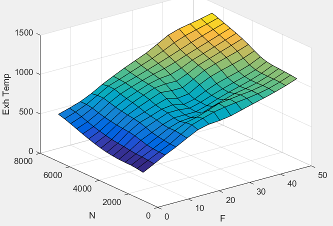Dependencies

To enable this parameter, for Torque model, select Simple Torque Lookup.

Engine load breakpoints used for exhaust temperature lookup table, in mg per injection.

Dependencies

To enable this parameter, for Torque model, select Simple Torque Lookup.

Engine speed breakpoints used for exhaust temperature lookup table, in rpm.

Dependencies

To enable this parameter, for Torque model, select Simple Torque Lookup.

Exhaust Temperature - Torque Structure

The optimal exhaust manifold gas temperature lookup table, ƒTexh, is a function of the engine speed engine speed and injected fuel mass, Texhopt = ƒTexh(F,N), where:

• Texhopt is optimal exhaust manifold gas temperature, in K.

• F is compression stroke injected fuel mass, in mg per injection.

• N is engine speed, in rpm.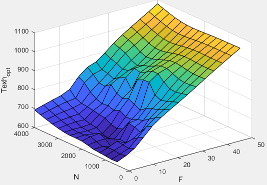Dependencies

To enable this parameter, for Torque model, select Torque Structure.

The main start of injection (SOI) timing exhaust temperature efficiency multiplier lookup table, ƒSOIexhteff, is a function of the engine speed engine speed and injected fuel mass, SOIexhteff = ƒSOIexhteff(ΔSOI,N), where:

• SOIexhteff is main SOI exhaust temperature efficiency multiplier, dimensionless.

• ΔSOI is main SOI timing relative to optimal timing, in degBTDC.

• N is engine speed, in rpm.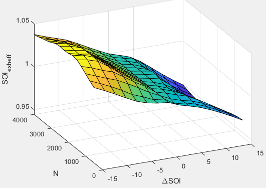Dependencies

To enable this parameter, for Torque model, select Torque Structure.

The intake manifold gas pressure exhaust temperature efficiency multiplier lookup table, ƒMAPexheff, is a function of the intake manifold gas pressure ratio relative to optimal pressure ratio and lambda, MAPexheff = ƒMAPexheff(MAPratio,λ), where:

• MAPexheff is intake manifold gas pressure exhaust temperature efficiency multiplier, dimensionless.

• MAPratio is intake manifold gas pressure ratio relative to optimal pressure ratio, dimensionless.

• λ is intake manifold gas lambda, dimensionless.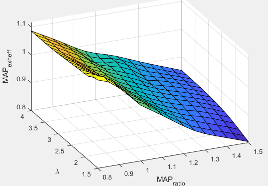Dependencies

To enable this parameter, for Torque model, select Torque Structure.

The intake manifold gas temperature exhaust temperature efficiency multiplier lookup table, ƒMATexheff, is a function of the engine speed and intake manifold gas temperature relative to optimal temperature, MATexheff = ƒMATexheff(ΔMAT,N), where:

• MATexheff is intake manifold gas temperature exhaust temperature efficiency multiplier, dimensionless.

• ΔMAT is intake manifold gas temperature relative to optimal temperature, in K.

• N is engine speed, in rpm.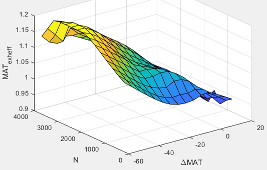Dependencies

To enable this parameter, for Torque model, select Torque Structure.

The intake manifold gas oxygen exhaust temperature efficiency multiplier lookup table, ƒO2Pexheff, is a function of the engine speed and intake manifold gas oxygen percent relative to optimal, O2Pexheff = ƒO2Pexheff(ΔO2P,N), where:

• O2Pexheff is intake manifold gas oxygen exhaust temperature efficiency multiplier, dimensionless.

• ΔO2P is intake gas oxygen percent relative to optimal, in percent.

• N is engine speed, in rpm.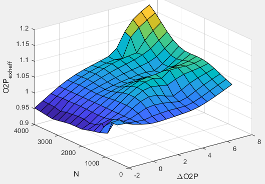Dependencies

To enable this parameter, for Torque model, select Torque Structure.

The fuel rail pressure efficiency exhaust temperature multiplier lookup table, ƒFUELPexheff, is a function of the engine speed and fuel rail pressure relative to optimal breakpoints, FUELPexheff = ƒFUELPexheff(ΔFUELP,N), where:

• FUELPexheff is fuel rail pressure exhaust temperature efficiency multiplier, dimensionless.

• ΔFUELP is fuel rail pressure relative to optimal, in MPa.

• N is engine speed, in rpm.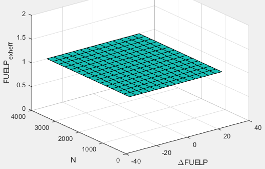Dependencies

To enable this parameter, for Torque model, select Torque Structure.

Post-injection cylinder wall heat loss transfer coefficient, in W/K.

Dependencies

To enable this parameter, for Torque model, select Torque Structure.

Emissions

The CI Core Engine CO2 emission mass fraction lookup table is a function of engine torque and engine speed, CO2 Mass Fraction = ƒ(Speed, Torque), where:

• CO2 Mass Fraction is the CO2 emission mass fraction, dimensionless.

• Speed is engine speed, in rpm.

• Torque is engine torque, in N·m.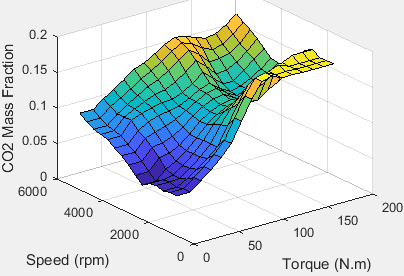Dependencies

To enable this parameter, on the Exhaust tab, select CO2.

The CI Core Engine CO emission mass fraction lookup table is a function of engine torque and engine speed, CO Mass Fraction = ƒ(Speed, Torque), where:

• CO Mass Fraction is the CO emission mass fraction, dimensionless.

• Speed is engine speed, in rpm.

• Torque is engine torque, in N·m.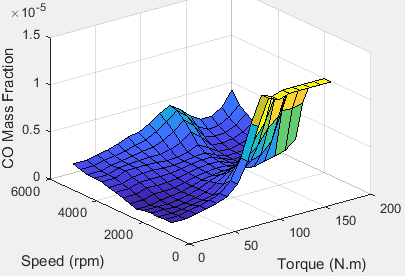Dependencies

To enable this parameter, on the Exhaust tab, select CO.

The CI Core Engine HC emission mass fraction lookup table is a function of engine torque and engine speed, HC Mass Fraction = ƒ(Speed, Torque), where:

• HC Mass Fraction is the HC emission mass fraction, dimensionless.

• Speed is engine speed, in rpm.

• Torque is engine torque, in N·m.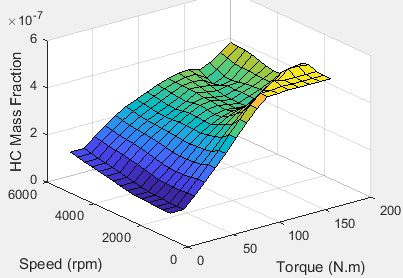Dependencies

To enable this parameter, on the Exhaust tab, select HC.

The CI Core Engine NOx emission mass fraction lookup table is a function of engine torque and engine speed, NOx Mass Fraction = ƒ(Speed, Torque), where:

• NOx Mass Fraction is the NOx emission mass fraction, dimensionless.

• Speed is engine speed, in rpm.

• Torque is engine torque, in N·m.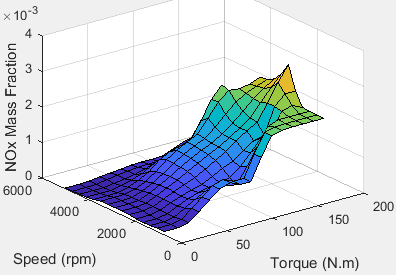Dependencies

To enable this parameter, on the Exhaust tab, select NOx.

The CI Core Engine PM emission mass fraction lookup table is a function of engine torque and engine speed where:

• PM is the PM emission mass fraction, dimensionless.

• Speed is engine speed, in rpm.

• Torque is engine torque, in N·m.

Dependencies

To enable this parameter, on the Exhaust tab, select PM.

Engine speed breakpoints used for the emission mass fractions lookup tables, in rpm.

Dependencies

To enable this parameter, on the Exhaust tab, select CO2, CO, NOx, HC, or PM.

Engine torque breakpoints used for the emission mass fractions lookup tables, in N·m.

Dependencies

To enable this parameter, on the Exhaust tab, select CO2, CO, NOx, HC, or PM.

Exhaust gas-specific heat, $C{p}_{exh}$, in J/(kg·K).

Fuel

Air-fuel ratio, $AFR$.

Fuel lower heating value, LHV, in J/kg.

Specific gravity of fuel, Sgfuel, dimensionless.

 Heywood, John B. Internal Combustion Engine Fundamentals. New York: McGraw-Hill, 1988.

Powertrain Blockset DocumentationGet trial now